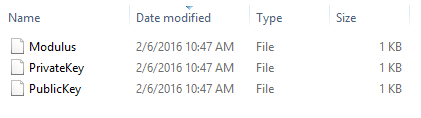## samedi 6 février 2016

### RSA with PowerShell - PowerRSA

Hi,

Based on this very good article (http://www.di-mgt.com.au/rsa_alg.html), I have implemented a RSA algorithm.

The code : https://github.com/giMini/PowerRSA/blob/master/PowerRSA.ps1

Caution : I did it for fun. Don't use it in production ;-) For the demo, I encrypt directly the data, in the real world, you encrypt the symetric key (which you exchange between Sender and Recipient) that will encrypt the data.

## Generate the keys

To generate the Modulus, the private key and the public key, enter this command

.\PowerRSA.ps1 -Method GenKeys

PowerRSA will generate the necessary keys
PS F:\Crypto> .\PowerRSA.ps1 -Method GenKeys
Keys generating...

Keys saved in F:\Crypto\20160206104626

and save them in a folderExample of the Modulus generatedTo generate a 2048-bit key :

.\PowerRSA.ps1 -Method GenKeys -KeyType 2048-bit

## How I do it

"This is the original algorithm.
1. Generate two large random primes, p and q, of approximately equal size such that their product n = pq is of the required bit length, e.g. 1024 bits.
2. Compute n = pq and (phi) φ = (p-1)(q-1).
3. Choose an integer e, 1 < e < phi, such that gcd(e, phi) = 1.
4. Compute the secret exponent d, 1 < d < phi, such that ed ≡ 1 (mod phi).
5. The public key is (n, e) and the private key (d, p, q). Keep all the values d, p, q and phi secret. [We prefer sometimes to write the private key as (n, d) because you need the value of n when using d. Other times we might write the key pair as ((N, e), d).]
• n is known as the modulus.
• e is known as the public exponent or encryption exponent or just the exponent.
• d is known as the secret exponent or decryption exponent."

For length k required of 2048 bits

1. I generate big (128 bytes) random number p and check if it is a Prime number with Rabbin-Miller algorithm
2. I generate big (128 bytes) random number q and check if it is a Prime number with Rabbin-Miller algorithm
3. I compute n (modulus) which is p * q
4. I compute φ (PHI) which is (p-1)*(q-1)
5. We choose 65537 or 10001 in Hex for Exponent Public Key(why ? In practice, common choices for e are 3, 5, 17, 257 and 65537 (216+1). These particular values are chosen because they are primes and make the modular exponentiation operation faster, having only two bits of value 1.
Aside: These five numbers are the first five Fermat numbers, referred to as F0 to F4, where Fx=2^(2^x)+1. Just be careful, these first five Fermat numbers are prime ("Fermat primes"), but the numbers F5 and above are not prime.
For example, F5 = 4294967297 = 641 × 6700417.
The usual choice for e is F4 = 65537 = 0x10001. Also, having chosen e, it is simpler to test whether gcd(e, p-1)=1 and gcd(e, q-1)=1 while generating and testing the primes in step 1. Values of p or q that fail this test can be rejected there and then. )
6.  I compute d which is the Exponent Private Key using the Extended Euclidean algorithm

## Encrypt data

To encrypt data using PowerRSA enter this command

.\PowerRSA.ps1 -Method Enc -Exponent F:\Crypto\20160206104626\PublicKey -Modulus F:\Crypto\20160206104626\Modulus

Enter the data string to encrypt :

Enter message to encrypt: Hi! I'm an encrypted data string

PowerRSA will encrypt data :
PS F:\Crypto> .\PowerRSA.ps1 -Method Enc -Exponent F:\Crypto\20160206104626\PublicKey -Modulus F:\Crypto\20160206104626\Modulus
Enter message to encrypt: Hi! I'm an encrypted data string :-)
Data saved in F:\Crypto\20160206110641

and save it in a folder
Encrypted data looks like that

## How I do it

"Sender A does the following:-

Obtains the recipient B's public key (n, e).
Represents the plaintext message as a positive integer m, 1 < m < n [see note 4].
Computes the ciphertext c = me mod n.
Sends the ciphertext c to B."

With the public key (which is composed of the Modulus and the Public Exponent), I encrypt the data string provided.

To crypt it and for each character:
1. I transform character into an integer representation
2. I generate a Random Padding
3. I concatenate the integer character representation with the random padding = m
4. I encrypt the concatenation result by compute c = me % n where c is the encrypted character, m = the concatenate integer character with random padding, e = the public Exponent key and n = the Modulus

## Decrypt data

To decrypt data using PowerRSA enter this command

.\PowerRSA.ps1 -Method Dec -Data F:\Crypto\20160206110641\Data -Exponent F:\Crypto\20160206104626\PrivateKey -Modulus F:\Crypto\20160206104626\Modulus

PowerRSA will decrypt the data :
PS F:\Crypto> .\PowerRSA.ps1 -Method Dec -Data F:\Crypto\20160206110641\Data -Exponent F:\Crypto\20160206104626\PrivateKey -Modulus F:\Crypto\20160206104626\Modulus
Hi! I'm an encrypted data string :-)

## How I do it

"Recipient B does the following:-

Uses his private key (n, d) to compute m = cd mod n.
Extracts the plaintext from the message representative m."

With the private key (which is composed of the Modulus and the Private Exponent), I decrypt the data string provided.
1. I decrypt the encoded string by compute m = cd % n
2. I remove the random padding
3. I transform the integer into its character representation